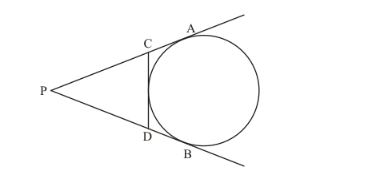# From an external point P, tangents PA and PB are drawn to a circle with centre O.

Question:

From an external point P, tangents PA and PB are drawn to a circle with centre O. If CD is the tangent to the circle at a point E and PA = 14 cm, find the perimeter of Δ PCD.

Solution:

Let us first put the given data in the form of a diagram.It is given that PA = 14cm. we have to find the perimeter of.

Perimeter ofis PC + CD + PD

Looking at the figure we can rewrite the equation as follows.

Perimeter ofis PC + CE + ED + PD ……(1)

From the property of tangents we know that the length of two tangents drawn to a circle from the same external point will be equal. Therefore,

CE =CA

ED =DB

Replacing the above in equation (1), we have,

Perimeter ofas PC + CA + DB + PD

By looking at the figure we get,

PC +CA =PA

DB +PD =PB

Therefore,

Perimeter ofis PA + PB

It is given that PA = 14 cm. again from the same property of tangents which says that the length of two tangents drawn to a circle from the same external point will be equal, we have,

PA = PB

Therefore,

Perimeter of= 2PA

Perimeter of= 2 × 14

Perimeter of= 28

Thus perimeter ofis 28 cm.